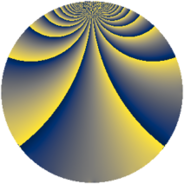# Properties

 Label 1470.2.uLevel $1470$ Weight $2$ Character orbit 1470.u Rep. character $\chi_{1470}(211,\cdot)$ Character field $\Q(\zeta_{7})$ Dimension $240$ Sturm bound $672$

# Related objects

## Defining parameters

 Level: $$N$$ $$=$$ $$1470 = 2 \cdot 3 \cdot 5 \cdot 7^{2}$$ Weight: $$k$$ $$=$$ $$2$$ Character orbit: $$[\chi]$$ $$=$$ 1470.u (of order $$7$$ and degree $$6$$) Character conductor: $$\operatorname{cond}(\chi)$$ $$=$$ $$49$$ Character field: $$\Q(\zeta_{7})$$ Sturm bound: $$672$$

## Dimensions

The following table gives the dimensions of various subspaces of $$M_{2}(1470, [\chi])$$.

Total New Old
Modular forms 2064 240 1824
Cusp forms 1968 240 1728
Eisenstein series 96 0 96

## Trace form

 $$240q - 4q^{3} - 40q^{4} - 4q^{7} - 40q^{9} + O(q^{10})$$ $$240q - 4q^{3} - 40q^{4} - 4q^{7} - 40q^{9} - 4q^{10} - 8q^{11} - 4q^{12} - 16q^{13} - 12q^{14} - 40q^{16} - 16q^{17} - 16q^{19} - 4q^{21} - 16q^{22} - 40q^{25} + 20q^{26} - 4q^{27} - 4q^{28} - 32q^{29} - 24q^{31} - 8q^{33} - 16q^{34} + 16q^{35} - 40q^{36} + 44q^{37} + 40q^{38} + 60q^{39} - 4q^{40} + 32q^{41} + 48q^{42} - 24q^{43} + 20q^{44} - 8q^{46} + 80q^{47} + 24q^{48} + 40q^{51} + 12q^{52} + 64q^{53} - 16q^{55} + 16q^{56} - 24q^{57} + 40q^{58} + 28q^{59} - 12q^{61} + 40q^{62} + 24q^{63} - 40q^{64} - 8q^{65} - 56q^{67} - 16q^{68} - 32q^{69} - 64q^{71} + 96q^{73} - 12q^{74} - 4q^{75} - 16q^{76} + 72q^{77} - 88q^{79} - 40q^{81} - 64q^{82} + 40q^{83} - 4q^{84} - 16q^{85} - 32q^{86} + 40q^{88} + 128q^{89} - 4q^{90} + 44q^{91} - 56q^{93} + 4q^{94} - 32q^{95} - 8q^{97} - 48q^{98} - 8q^{99} + O(q^{100})$$

## Decomposition of $$S_{2}^{\mathrm{new}}(1470, [\chi])$$ into newform subspaces

The newforms in this space have not yet been added to the LMFDB.

## Decomposition of $$S_{2}^{\mathrm{old}}(1470, [\chi])$$ into lower level spaces

$$S_{2}^{\mathrm{old}}(1470, [\chi]) \cong$$ $$S_{2}^{\mathrm{new}}(49, [\chi])$$$$^{\oplus 8}$$$$\oplus$$$$S_{2}^{\mathrm{new}}(98, [\chi])$$$$^{\oplus 4}$$$$\oplus$$$$S_{2}^{\mathrm{new}}(147, [\chi])$$$$^{\oplus 4}$$$$\oplus$$$$S_{2}^{\mathrm{new}}(245, [\chi])$$$$^{\oplus 4}$$$$\oplus$$$$S_{2}^{\mathrm{new}}(294, [\chi])$$$$^{\oplus 2}$$$$\oplus$$$$S_{2}^{\mathrm{new}}(490, [\chi])$$$$^{\oplus 2}$$$$\oplus$$$$S_{2}^{\mathrm{new}}(735, [\chi])$$$$^{\oplus 2}$$